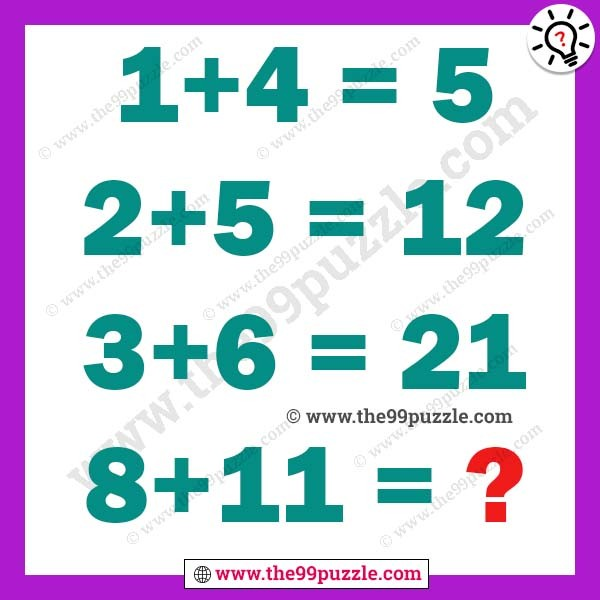# Viral Math Puzzle that Stumps on the Internet – Puzz101

Can you solve the Viral Math Puzzle that Stumps on the Internet? In this math, puzzles have various answers in various methods.

1+4=5

2+5=12

3+6=21

8+11=?###### Explanation:

There’s three different way to calculate the answer.

Method I.

1 + 4 = 1 + 1(4) = 5

2 + 5 = 2 + 2(5) = 12

3 + 6 = 3 + 3(6) = 21

8 + 11 = 8 + 8(11) = 96

Now, there is another method to calculate the answer by summing up the previous summation result with the digits.

Method II.

1 + 4 = 5
5 + 2 + 5 = 12
12 + 3 + 6 = 21
21 + 4 + 7 = 32
32 + 5 + 8 = 45
45 + 6 + 9 = 60
60 + 7 + 10 = 77
77 + 8 + 11 = 96

Method III.

1 + 4 = 5

5 + 2 + 5 = 12

12 + 3 + 6 = 21

21 + 8 + 11 = 40